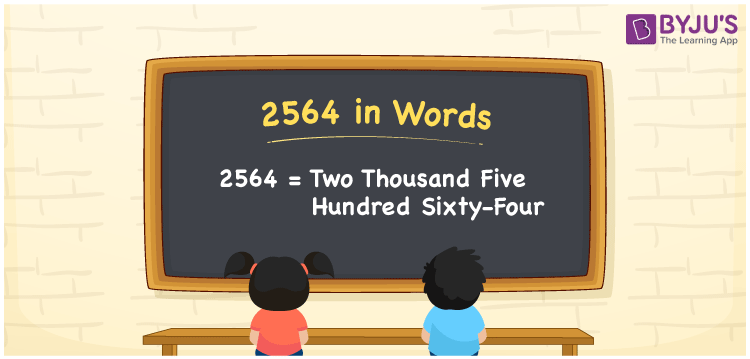# 2564 in Words

We can write 2564 in words as Two thousand five hundred sixty-four. If your monthly expense on milk is Rs. 2564, then you can say, “My monthly expenditure on milk is Two thousand five hundred sixty-four rupees”. Thus, Two thousand five hundred sixty-four is the number name for 2564. In this article, you will learn how to convert the cardinal number 2564 into words effortlessly.

 2564 in words Two thousand five hundred sixty-four Two thousand five hundred sixty-four in Numbers 2564

## 2564 in English Words

We generally write numbers in words using the English alphabet. So, we express 2564 in English words as “Two thousand five hundred sixty-four”.## How to Write 2564 in Words?

The number 2564 contains four digits so let’s make a place value chart with four columns, as given below.

 Thousands Hundreds Tens Ones 2 5 6 4

Here, ones = 4, tens = 6, hundreds = 5, and thousands = 2.

By expanding the above numbers, we get;

2 × Thousand + 5 × Hundred + 6 × Ten + 4 × One

= 2 × 1000 + 5 × 100 + 6 × 10 + 4 × 1

= 2000 + 500 + 60 + 4

= Two thousand + Five hundred + Sixty + Four

= Two thousand five hundred sixty-four

Therefore, 2564 in words = Two thousand five hundred sixty-four.

As we know, 2564 is a natural number that precedes 2565 and succeeds 2563.

2564 in words – Two thousand five hundred sixty-four

Is 2564 an odd number? – No

Is 2564 an even number? – Yes

Is 2564 a prime number? – No

Is 2564 a composite number? – Yes

Is 2564 a perfect square number? – No

Is 2564 a perfect cube number? – No

## Frequently Asked Questions on 2564 in Words

Q1

### Write 2564 in words.

We can write the number 2564 in words as Two thousand five hundred sixty-four.
Q2

### How to write Rs. 2564 in words on a cheque?

On a cheque, we generally write Rs. 2564 in words as “Two thousand five hundred sixty-four rupees only”.
Q3

### What number is Two thousand five hundred sixty-four in words?

The number 2564 is written as Two thousand five hundred sixty-four in words.